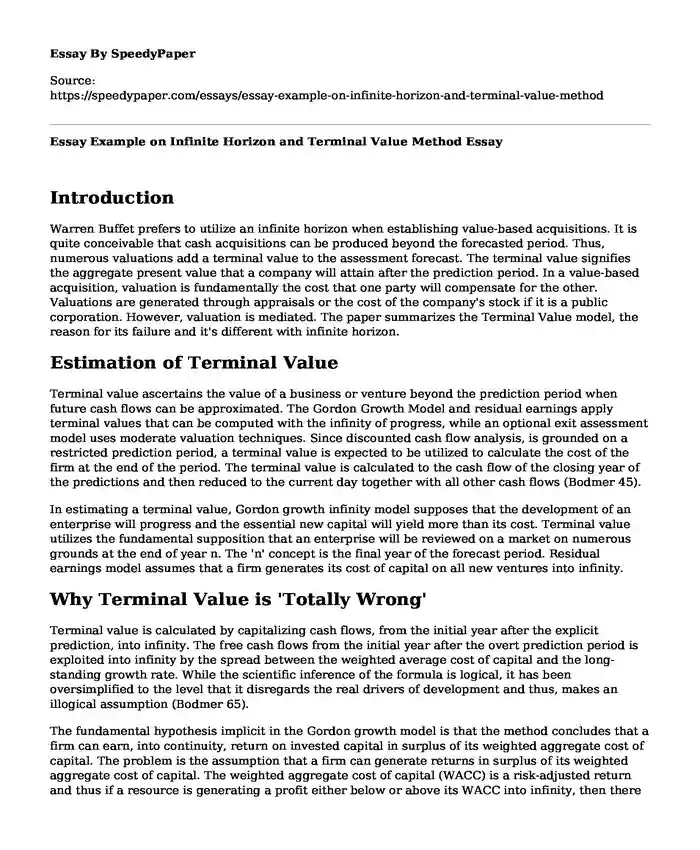# Essay Example on Infinite Horizon and Terminal Value Method

Published: 2022-12-26Type of paper: Essay Categories: Audit Accounting Pages: 4 Wordcount: 858 words
143 views

## Introduction

Warren Buffet prefers to utilize an infinite horizon when establishing value-based acquisitions. It is quite conceivable that cash acquisitions can be produced beyond the forecasted period. Thus, numerous valuations add a terminal value to the assessment forecast. The terminal value signifies the aggregate present value that a company will attain after the prediction period. In a value-based acquisition, valuation is fundamentally the cost that one party will compensate for the other. Valuations are generated through appraisals or the cost of the company's stock if it is a public corporation. However, valuation is mediated. The paper summarizes the Terminal Value model, the reason for its failure and it's different with infinite horizon.

Is your time best spent reading someone else’s essay? Get a 100% original essay FROM A CERTIFIED WRITER!

## Estimation of Terminal Value

Terminal value ascertains the value of a business or venture beyond the prediction period when future cash flows can be approximated. The Gordon Growth Model and residual earnings apply terminal values that can be computed with the infinity of progress, while an optional exit assessment model uses moderate valuation techniques. Since discounted cash flow analysis, is grounded on a restricted prediction period, a terminal value is expected to be utilized to calculate the cost of the firm at the end of the period. The terminal value is calculated to the cash flow of the closing year of the predictions and then reduced to the current day together with all other cash flows (Bodmer 45).

In estimating a terminal value, Gordon growth infinity model supposes that the development of an enterprise will progress and the essential new capital will yield more than its cost. Terminal value utilizes the fundamental supposition that an enterprise will be reviewed on a market on numerous grounds at the end of year n. The 'n' concept is the final year of the forecast period. Residual earnings model assumes that a firm generates its cost of capital on all new ventures into infinity.

## Why Terminal Value is 'Totally Wrong'

Terminal value is calculated by capitalizing cash flows, from the initial year after the explicit prediction, into infinity. The free cash flows from the initial year after the overt prediction period is exploited into infinity by the spread between the weighted average cost of capital and the long-standing growth rate. While the scientific inference of the formula is logical, it has been oversimplified to the level that it disregards the real drivers of development and thus, makes an illogical assumption (Bodmer 65).

The fundamental hypothesis implicit in the Gordon growth model is that the method concludes that a firm can earn, into continuity, return on invested capital in surplus of its weighted aggregate cost of capital. The problem is the assumption that a firm can generate returns in surplus of its weighted aggregate cost of capital. The weighted aggregate cost of capital (WACC) is a risk-adjusted return and thus if a resource is generating a profit either below or above its WACC into infinity, then there are high chances of mispricing (inappropriate valuation) of the asset. In another viewpoint, when a firm is generating revenues in surplus of its WACC, then rival corporations will enter into the specific market and ultimately drive down surplus revenues until profits generated by the firm approximate its cost of capital (Bodmer 67).

## Contrast with 'Infinite Horizon'

Infinite horizon is a valuation without a specific point in time when all ventures must be liquidated and returns have an immediate utilization. The method ensures that entrepreneurs retain and advance their fortunes over numerous generations. As much as wealth generation is an integration of competence, hard work, and luck, maintaining wealth is a time-testing process that can be continuously be repeated over generations. In investing in value-based acquisition using infinite horizon, Buffet assumes that the assets are able to retain their wealth over time and increase their value over time. Infinite horizon assumes that the company has a finite lifespan and that it will be liquidated at the end of that life (Baranowski).

In contrast, the Terminal Value model uses certain average assumptions to calculate the value of the company beyond the prediction period. In this model, the lifespan of a company is projected by the entrepreneur. It can be two or ten years. Terminal Value method is used in financial modeling to decide the percentage of the project value in a discounted cash flow valuation. In this model, after the completion of the forecast period, the returns can be reinvested back into new assets and prolong lives.

## Conclusion

Conclusively, it is clear why Warren Buffet uses infinite horizon method in value-based acquisition instead of terminal value model. Infinite horizon assures that the firm will last for generations and will continue to build up its value. In the terminal value model, a business lasts a duration desired by the owner. After the end of that period, the owners take the returns and invest in other ventures. Terminal value is totally wrong as it increases the chance of mispricing of assets. It also disregards the real drivers of growth thus making an irrational assumption.

## Works Cited

Baranowski, Bogumil. Infinite Horizon, Finite Assets. (2018). https://moiglobal.com/infinite-horizon-finite-assets/. Accessed: 25 April 2019.

Bodmer, Edward. Corporate and Project Finance Modeling: Theory and Practice. , 2015. Print.

Essay Example on Infinite Horizon and Terminal Value Method. (2022, Dec 26). Retrieved from https://speedypaper.com/essays/essay-example-on-infinite-horizon-and-terminal-value-method

Request Removal

If you are the original author of this essay and no longer wish to have it published on the SpeedyPaper website, please click below to request its removal:

Liked this essay sample but need an original one?

Hire a professional with VAST experience!